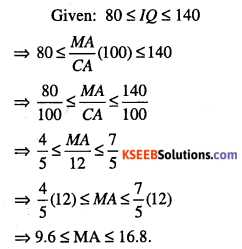# 1st PUC Maths Question Bank Chapter 6 Linear Inequalities

Students can Download Maths Chapter 6 Linear Inequalities Questions and Answers, Notes Pdf, 1st PUC Maths Question Bank with Answers helps you to revise the complete Karnataka State Board Syllabus and score more marks in your examinations.

## karnataka 1st PUC Maths Question Bank Chapter 6 Linear Inequalities

Question 1.
Define inequality.
Two real numbers or two algebraic expressions related by the symbol ‘<’, V, ‘<’ or ‘>’ form an inequality.
For example: 3 < 5, 7 > 5 are numerical inequalities x < 5,y > 2, x > 3 are literal inequalities. ax + b < 0, ax + b > 0 are strick inequalities in one variable.
ax + b < 0, ax + b >0 are slack inequalities in one variable.
ax + by < c, ax + by > c are strick inequalities in two variables.
ax + by < c, ax + by > c are slack inequalities in two variables.
ax2 + bx + c < 0, ax2 + bx + c > 0 are quadratic inequalities in one variable.

Question 2.
Define solution set.
The set of all those values of x which satisfy the given inequation is called the solution set of inequation.

Rules for solving inequations:

• Rule 1: Adding the same number or expression to each side of an inequation docs not change the inequality.
• Rule 2: Subtracting the same number or expression from each side of an inequation does not change the inequality.
• Rule 3: Multiplying (or dividing) each side of an inequation by the same positive number does not change the inequality.
• Rule 4: Multiplying (or dividing) each side of an inequation by same negative number reverses the inequality.

Inequality solver is an application help you to solve linear inequality and tracing linear equations and root point.Question 3.
Solve 30x < 200 when
(i) x is a natural number
(ii) x is an integer.
Given: 30x < 200
$$x<\frac{200}{30}=6.6$$

(i) x is a natural number:
Solution set = { 1, 2, 3,4, 5, 6}

(ii) x is a real number:
∴ Solution set = {… -3, -2, -1,0, 1,2, 3,4, 5, 6}

Question 4.
Solve 24x < 100, when
(i) x is a natural number
(ii) x is an integer.
Given: 24x < 100
$$x<\frac{100}{24}=4.16$$
(i) when x∈N, solution set ={ 1, 2, 3, 4}
(ii) when x∈Z,
∴ Solution set= {… -3, -2, -1, 0,1, 2, 3, 4}

Question 5.
Solve -12x > 30, when
(i) x∈N
(ii) x∈Z
Given: -12x > 30
$$\Rightarrow x<\frac{30}{-12}=-2.5$$
(i) When x∈N, solution set = φ
(ii) When x∈Z, solution set ={…, -5, -4, -3}

Question 6.
Solve: 5x – 3 < 7, when
(i) x is an integer
(ii) x is a real number.
Given: 5x – 3 < 7
⇒ 5x<7 + 3 = 10
∴ x< $$\frac{10}{5}=2$$
(i) If x is an integer,
solution set = {…, -3, -2, -1, 0, 1}.
(ii) If x is a real number, solution set = (-∞, 2).

Question 7.
Solve: 3x + 8 > 2, when
(i) x is an integer
(ii) x is a real number.
Given: 3x + 8 > 2
⇒ 3x > 2 – 8 = – 6
∴ x>-$$\frac{6}{3}=-2$$
(i) When x∈Z, solution set = {-1, 0, 1, 2,3,…}
(ii) When x∈M, solution set = (-2,∞).

Question 8.
Solve: 5x – 3 < 3x + 1 when
(i) x is an integer
(ii) x is a real number.
Given: 5x – 3 < 3x + 1
⇒ 5x – 3x <1 + 3
⇒ 2x<4
$$\Rightarrow x<\frac{4}{2}=2$$
(i) When x∈Z, solution set={…, -3,-2,-1, 0,1}
(ii) When x ∈M, solution set = (- ∞, 2).

Question 9.
Solve: 4x + 3 < 6x + 7.
Given: 4x + 3 < 6x + 7
⇒ -7 + 3 < 6x – 4x
⇒ -4 <2x
⇒ $$\Rightarrow x>\frac{-4}{2}=-2$$
∴ Solution set = (-2, ∞).

Question 10.
Solve: 4x + 3 < 5x + 7
Given: 4x + 3 < 5x + 7
⇒ -7 + 3 < 5x – 4x
⇒ -4 < x
∴ Solution set = (-4, ∞).

Question 11.
Solve: 3x – 7 > 5x -1
Given: 3JC – 7 > 5x – 1
⇒1 – 7 > 5x – 3x
⇒-6 > 2x
∴ ⇒ x>$$\frac{-6}{2}=-3$$
∴  Solution set = (-3, ∞)

Question 12.
Solve: 3(x – 1) < 2 (^ – 3)
Given: 3(x – 1) < 2 (x – 3)
⇒3x-3<2x-6
⇒ 3x – 2x < -6 + 3
⇒x<-3
∴  Solution set = (-∞,-3].Question 13.
Solve: 3(2 – x) > 2(1 – x)
Given 6 – 3x > -2x + 3x
⇒ 6 – 2 > -2x + 3x
⇒ 4>x.
∴ Solution set = (-∞, 4]

Question 14.
Solve:
$$x+\frac{x}{2}+\frac{x}{3}<11$$Question 15.
Solve:
$$\frac{x}{3}>\frac{x}{2}+1$$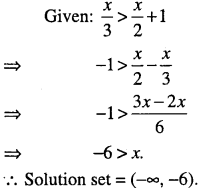Question 16.
Solve:
$$\frac{5-2 x}{3} \leq \frac{x}{6}-5$$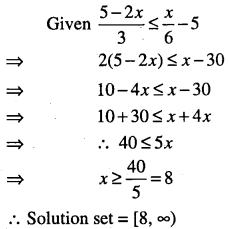Question 17.
Solve:
$$\frac{3(x-2)}{5} \leq \frac{5(2-x)}{3}$$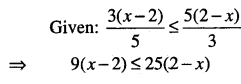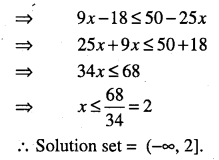Question 18.
Solve:
$$\frac{1}{2}\left(\frac{3 x}{5}+4\right) \geq \frac{1}{3}(x-6)$$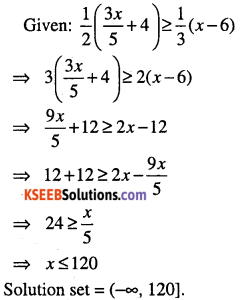Question 19.
Solve: 2(2x+ 3) -10 < 6(x -2)
Given: 4x + 6-10<6x-12
⇒ 12-4<6x-4x
⇒ 8 < 2x
$$\Rightarrow \quad x>\frac{8}{2}=4$$
∴ Solution set = (4, ∞)Question 20.
Solve: 37 – (3x+ 5) ≥ 9x – 8(x – 3)
Given: 37 – (3x+ 5) ≥ 9x – 8(x – 3)
⇒ 32 – 24 ≥ x + 3x
⇒ 8 >4x
⇒x<2
∴  Solution set = (-∞,2)

Question 21.
Solve:
$$\frac{x}{4}<\frac{5 x-2}{3}-\frac{7 x-3}{5}$$
$$\frac{x}{4}<\frac{5(5 x-2)-3(7 x-3)}{15}-\frac{4 x-1}{15}$$
⇒15x<16x-4
⇒ 4<16x-15x
⇒ x>4
∴ Solution set = (4, ∞).           .

Question 22.
Solve:
$$\frac{2 x-1}{3} \geq \frac{3 x-2}{4}-\frac{2-x}{5}$$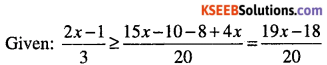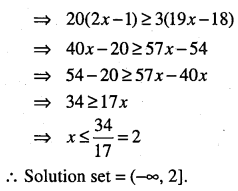Question 23.
Solve the following inequalities and show the graph of the solution in each case on number line
(i) 3x – 2 < 2x +1
(ii) 5x – 3 > 3x- 5
(iii) 7x + 3 < 5x + 9
(iv) 3(1-x)<2(x + 4)
(v) $$\frac{3 x-4}{2} \geq \frac{x+1}{4}-1$$
(vii) $$\frac{x}{2} \geq \frac{5 x-2}{3}-\frac{7 x-3}{5}$$
(i) Given: 3x – 2 < 2x +1
⇒ 3x-2x<1 + 2
⇒ x < 3
∴ Solution set = (-<», 3)
The graph of the solution set is given belowQuestion 24.
The marks obtained by a student of class XI in first and second terminal examination are 62 and 48, respectively. Find the minimum marks he should get in the annual examination to have an average of at least 60 marks.
Let x be the marks obtained by student in the annual examination.
Then
\begin{aligned} &\frac{62+48+x}{3} \geq 60\\ &\Rightarrow 110+x \geq 180\\ &\Rightarrow x \geq 70 \end{aligned}
Thus, the student must obtain a minimum of 70 marks to get an average of at least 60 marks.Question 25.
Ravi obtained 70 and 75 marks in first two unit test. Find the minimum marks he should get in the third test to have an average of at least 60 marks.
Let x be the marks obtained in the third test.
$$\frac{70+75+x}{3} \geq 60$$
⇒ 145+ x≥180
⇒ x> 180-145 = 35.
Thus, Ravi must obtain a minimum of 35 marks to get an average of atleast 60 marks.

Question 26.
To receive grade ‘A’ in a course, one must obtain an average of 90 marks or more in five examinations (each of 100 marks). If Sunita’s marks in first four examinations are 87, 92, 94 and 95, find minimum marks that Sunita must obtain in fifth examination to get grade ‘A’ in the course.
Let x be the marks in the fifth test obtained by Sunita
$$\frac{87+92+94+95+x}{5} \geq 90$$
⇒ 368 + x>450⇒x>82
So, Sunita must obtain a minimum of 82 marks to get grade ‘A’.

Question 27.
Find all pairs of consecutive odd natural numbers, both of which are larger than 10, such that their sum is less than 40.
Let x be the smaller of the two consecutive odd natural number, so that the other one is x + 2.
Given x> 10 and x + (x + 2) < 40
⇒ 2x < 38
⇒ x<19
∴10 <x< 19
Since x is an odd number, x can take the values 11,13, 15 and 17.
∴ Required possible pairs are
(11,13), (13,15), (15, 17), (17,19).

Question 28.
Find all pairs of consecutive odd positive integers both of which are smaller than 10 such that their sum is more than 11.
Let x and x + 2 be the required pairs of consecutive odd positive integers.
Given: x + 2<10
⇒ x<8 and
⇒ x + x + 2>11
⇒ 2x>9
⇒ x > 4.5
∴ 4.5 < x < 8
x = 5, 7
∴ Required possible pairs are (5, 7), (7, 9).Question 29.
Find all pairs of consecutive even positive integers, both of which are larger than 5 such that their sum is less than 23.
Let x and x + 2 be the required pairs of consecutive even positive integers.
Given: x > 5
x + x + 2 <23
⇒ 2x< 21
⇒ x< 10.5
∴ 5 < x < 10.5
x = 6, 8, 10
∴ Required possible pairs are (6, 8), (8, 10), (10,12)

Question 30.
The longest side of a triangle is 3 times the shortest side and the third side is 2 cm shorter then the longest side. If the perimeter of the triangle is at least 61 cm, find the minimum length of the shortest side.
Let x, 3x, 3x – 2 be the sides of a triangle
Given: x + 3x + 3x – 2 ≥ 61
7x ≥63
x ≥ 9.
∴ Minimum length of shortest side = 9 cm.
∴ Other sides are 27 and 25.

Question 31.
A man wants to cut three lengths from a single piece of board of length 91 cm. The second length is to be 3 cm longer than the shortest and the third length is to be twice as long as the shortest. What are the possible lengths of the shortest board if the third piece is to be at least 5 cm longer than the second?
Let x be the length of the shortest board then
x + 3 and 2x are the lengths of the second and third piece, respectively.
⇒ x+(x+3) + 2x≤91 and 2x≥(x + 3) + 5
4x≤88 and x≥8
⇒ x≤22 and x ≥ 8
⇒ 8 ≤ x ≤22.
∴ The possible length of board is at least 8 cm long but not more than 22 cm long.Question 32.
Solve the following inequalities graphically in two dimensional plane:
(i) x > -3
(ii) y < -2
(iii) x + y< 5
(iv) 2x – 3y > 6
(v) 3x + 2y > 6
(vi) 3y – 5x < 30
(vii) y>2
(viii) 3x – 6 > 0
(ix) 2x + y > 6
(x) 3x + 4y < 12
(xi) y + 8 > 2x
(xii) x-y <2
(xiii) -3x + 2y > -6
(i) Consider the equation x = -3.
Draw a graph of x = -3 by dotted line, which is parallel to y-axis, to the left of y-axis at distance
Put x = 0 in the given inequation x > -3, we get 0 > -3 which is true.
∴ Solution region consists of (0, 0).∴ The shaded region is the solution region

(ii) y < -2 ………….(1)
Consider the equation y = -2.
Draw a graph of y = -2 by dotted line, which is parallel to x-axis, below x-axis and at distance 2. Put y = 0 in (1), 0 < -2 which is not true.
∴ Solution region is not containing (0, 0).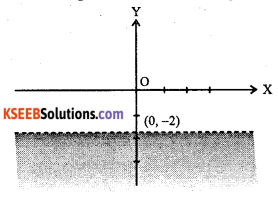∴ Shaded region is the solution region.

(iii) x + y < 5  ………….(1)
Draw the graph of x + y = 5 by dotted line.
Points (5, 0) and (0, 5) lie on it. Join these points.
Put x = 0 and y = 0 in (1), we get 0 + 0 < 5 which is true.
∴ The solution region contains the origin.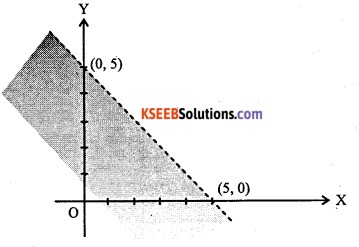∴ The shaded region is the solution region

(iv) 2x- 3y > 6  ………….(1)
Draw the graph of 2x – 3y = 6 by dotted line It passes through (3, 0) and (0, -2). Join these points.
Put x = 0 and y = 0 in (1), we get 0 – 0 > 6 which is not true.
∴ Solution region does not contain the origin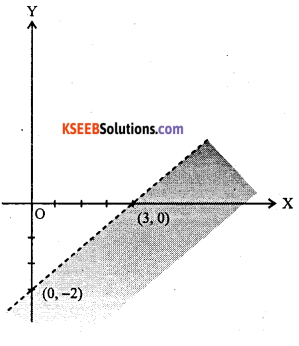∴ Shaded region is the solution region

(v) 3x + 2y > 6 ………….(1)
Draw the graph of 3x + 2y = 6 by dotted line.
It passes through (2, 0) and (0, 3). Join these points.
Put x = 0 and y = 0 in (1), we get 0 + 0 > 6 which is not true.
∴ The solution region does not contain the origin.∴ The shaded region is the solution region,(vi) 3y- 5x< 30 …(1)
Draw the graph of 3y – 5x = 30 by dotted line.
It passes through (-6,0) and (0,10)
Join these two points put x = 0 and y = 0 in (1),
we get 0 – 0 < 30 which is true.
∴ The solution region contains the origin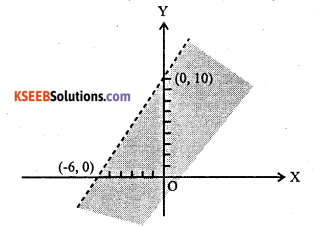The shaded region is the solution region

(vii) y > 2 ………………(1)
Draw the graph of y = 2 by dotted line Put y = 0 in (1)
we get 0 > 2 which is false.
∴ Solution region doesn’t contain the origin.∴ The shaded region is the solution region.

(viii) 3x- 6 ≥ 0 ………………..(1)
Draw the graph of 3x -6 = 0 i.e., x = 2 by thick line
Put x = 0 in (1), we get -6 > 0 which is false.
∴ Solution region does not contains the originThe shaded region is the solution region,

(ix) 2x + y >6 …………………..(1)
Draw the graph of 2x + y = 6 by thick line.
It passes through (3, 0) and (0, 6).
Join these points, put x = 0 and y = 0 in (1), we get 0 + 0 > 6 which is false.
∴ Solution region does not contain the origin(x) 3x + 4y < 12 …………… (1)
Draw the graph of 3x + 4y = 12 by thick line.
It passes through (4, 0) and (0, 3).
Join these points, put x = 0 and y = 0 in (1), we get 0 + 0 ≤ 12 which is true.
∴ The solution region contains the origin.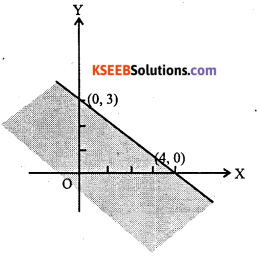∴ The shaded region is the solution region,

(xi) y + 8 ≥2x ………… (1)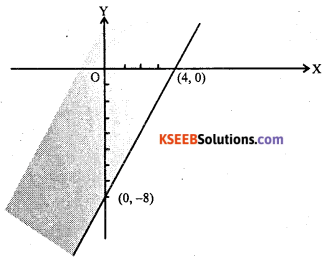Draw the graph of y+8 = 2 by thick line
It passes through (4, 0) and (0, -8)
Join these points, put x = 0 and y = 0 in (1), we get 0+8≤0 which is true.
∴ The solution region contains the origin
∴ The shaded region is the solution region,

(xii)  x – y ≤ 2 ………… (1)
Draw the graph of x – y ≤ 2 by thick line
It passes through (2, 0) and (0, -2)
Join these points, put x = 0 and y = 0 in (1), we get 0 – 0 < 2 which is true.
∴ The solution region contains the origin∴ The shaded region is the solution region.

(xiii) -3x + 2y>-6 ………… (1)
Draw a graph of -3x + 2y = -6 by thick line.
It passes through (2, 0) and (0, -3)
Join these points, put x = 0 and y = 0 in (1), we get 0 + 0 > -6 which is true.
∴ The solution region contains the origin∴ The shaded region is the solution region.

Question 33.
Solve the following system of inequalities graphically.
(j) x≥3,y≥2
(ii) x+y≥5,x-y≤3(i) x≥3 ……………………… (1)
First draw the line x = 3 by thick line.
Put x = 0 in (1), we get 0 > 3 is not true.
∴ Solution set of (1) is not containing the origin.
y≥2 ………………….. (2)
Now draw the line y = 2 by thick line Put y = 0 in (2), we get, 0 > 2 is not true.
∴ Solution set of (2) is not containing the origin.
∴ Shaded region is the required solution region.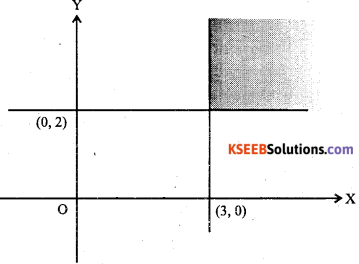(ii) Consider x + 3 ≥ 5 ……………….. (1)
Draw the graph of x + y = 5 by thick line.
It passes through (5, 0) and (0, 5)
Join these points put x = 0 and y = 0 in (1), we get 0 + 0 ≥ 5 which is false
∴ Solution set of (1) is not containing the origin.

Consider x-y ≤3  ……………….. (2)
Draw the graph of x – y = 3 by thick line It passes through (3,0) and (0, -3)
Join these points, put x = 0 and y = 0 in (2), we get 0 – 0 < 3 which is true.
∴ Solution set of (2) is containing the origin.
Hence, the shaded region represents the solution set of the given system of inequalities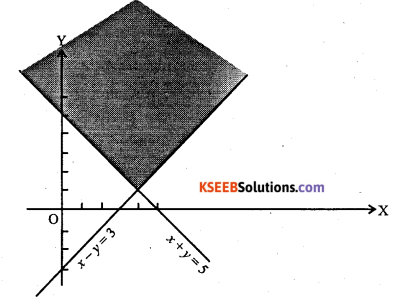(iii) Consider 2x + y ≥ 6  ……………….(1)
Draw the graph of 2x + y = 6 i.e. $$\frac{x}{3}+\frac{y}{6}=1$$by thick line.
It passes through (3, 0) and (0, 6).
Join these points put x = 0 and y = 0 in (1), we get 0 + 0 ≥ 6 which is false.
∴ Solution set of (1) is not containing the origin.
Consider 3x+4y ≤12  ……………….. (2)
Draw the graph of 3x+4y ≤12 i.e
$$\frac{x}{4}+\frac{y}{3}=1$$by thick line.

It passes through (4, 0) and (0, 3).
Join these points put x = 0 and y = 0 in (2), we get 0 + 0 < 12 which is true.
∴ Solution set of (2) is containing the origin.
Hence, the shaded region represents the solution set of the given system of inequalities.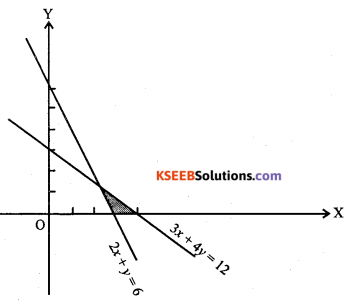(iv) Consider x+y≥4 ………………(1)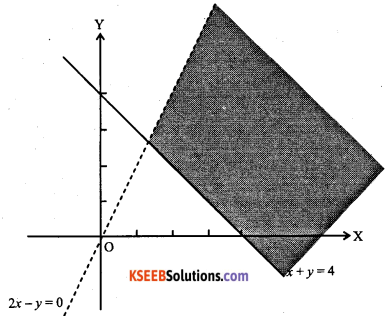Draw the graph of x+y=4 by thick line.
It passes through (4, 0) and (0,4)
Join these points put x = 0 and y = 0 in (1), we get 0 + 0 > 4 which is false.
∴ Solution set of (1) is not containing the origin.
Consider 2x – y > 0 ………………(2)
Draw the graph of 2x – y = 0 by dotted line.
It passes through (0, 0) and (1,2)
Join these points put x = 2 and y = 1 in (2),
we get
2(2) – 1 > 0 which is true.
∴ Solution set of (2) is containing the point (2, 1)
Hence, the shaded region represents the solution set of the given system of inequations.(v) Consider 2x -y > 1 ………………(1)
Draw the graph of 2x – y = 1 by dotted line
It passes through $$\left(\frac{1}{2}, 0\right)$$[ and (0,-1).
Join these points, put x = 0 and y = 0 in (1), we get 0 – 0 > 1 which is false.
∴ Solution set of (1) is not containing the origin.
Consider x – 2y < -1 ……………… (2)
Draw the graph of x – 2y = -1 by dotted line.
It passes through (-1, 0) and $$(-1,0) \text { and }\left(0, \frac{1}{2}\right)$$
Join these points, put x = 0 and y = 0 in (2) we get 0 – 0 < -1 which is false.
∴ Solution set of (2) is not containing the origin.
Hence, the shaded region represents the solution set of the given system of inequations.(vi) Consider x+y≤6 ………………(1)
Draw the graph of x + y = 6 by thick line. It passes through (6, 0) and (0, 6)
Join these points put x = 0 and y = 0 in (1), we get 0 + Q < 6 which is true.
∴ Solution set of (1) is containing the origin.
Consider x + y ≥ 4  ……………… (2)
Draw the graph of x + y = 4 by thick line It passes through (4, 0) and (0, 4).
Join these points, put x = 0 and y = 0 in (2), we get 0 + 0 ≥ 4 which is false
∴ Solution set of (2) is not containing the origin.
Hence, the shaded region represents the solution set of the given system of inequations.(vii) Consider 2x+y≥8 ……………… (1)
Draw the graph 2x + y = 8 by thick line.
It passes through (4, 0) and (0, 8)
Join these points, put x = 0 and y = 0 in (1), we get 0 + 0 ≥ 8 which is false
∴ Solution set of (1) is not containing the origin.
Consider x + 2y > 10 ……………… (2)
Draw the graph x + 2y = 10 by thick line It passes through (10, 0) and (0, 5).
Join these points, put x = 0 and y = 0 in (2), we get 0 + 0 > 10 which is false
Solution set of (2) is not containing the origin.
Hence, the shaded region represents the solution set of the given system of inequations.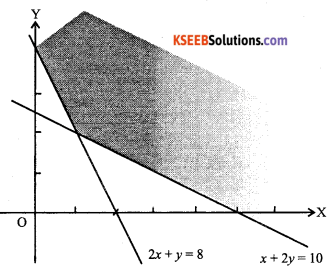(viii) Consider 8x + 3y ≤100 ……………… (1)
Draw the graph of 8x + 3y = 100 by thick line
It passes through $$\left(\frac{25}{2}, 0\right) \text { and }\left(0, \frac{100}{3}\right)$$
Join these points, put x = 0 and y = 0 in (1),
we get 0+0 ≤ 100 which is true.
∴ Solution set of (1) contains the origin.
Since x ≥0, y ≥0, every point in the shaded region in the first quadrant, including the points on the line and the axes, represents the solution of the given system of inequations.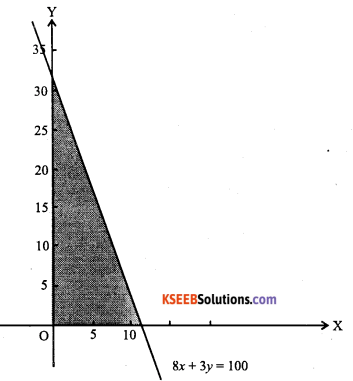(ix) Consider 5x + 4y ≤ 40 ………………… (1)
Draw the graph of 5x + 4.y = 40 by thick line.
It passes through (8, 0) and (0, 10).
Join these points, put x = 0 and y = 0 in (1), we get 0 + 0 ≤ 40 which is true.
Solution set of (1) contains the origin.
Consider x ≥ 2 ………………… (2)
Draw the graph of x= 2.
Clearly solution set of (2) is not containing the origin.
Consider y ≥ 3  ………………… (3)
Draw the graph of y = 3
Clearly solution set of (3) is not containing the origin.
Hence, the shaded region represents the solution set of the given system of inequations.(x) Consider 3x + 2y≤12 ………………… (1)
Draw the graph of 3x + 2y = 12 by thick line.
It passes through (4, 0) and (0, 6).
Join these points put x = 0 and y = 0 in (1), we get
0+0≤12 which is true.
∴ Solution set of (1) contains the origin
Consider x ≥1 …………….. (2)
Draw the graph of x= 1
Clearly solution set of (2) is not containing the origin.
Consider y ≥ 2 … (3)
Clearly solution set of (3) is not containing the origin.
Hence, the shaded region represents the solution set of the given system of inequations.(xi) Consider 5x+4y≤20 …………(1)
Draw the graph of 5x+4y = 20 by thick line
It passes through (4, 0) and (0, 5).
Join these points, put x = 0 and y = 0 in (1), we get 0 + 0 < 20 which is true.
∴ Solution set of (1) contains the origin. Consider x > 1 …………….. (2)
Draw the graph of x = 1 clearly solution set of (2) is not containing the origin.
Consider y > 2 …………….. (3)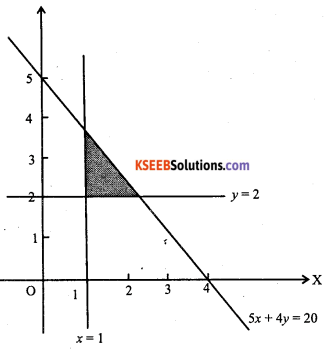Draw the graph of y = 2 clearly solution set of (3) is not containing the origin.
Hence, the shaded region represents the solution set of the given system of inequations.

(xii) Consider x + y ≤ 9 …………….. (1)
Draw the graph of x + y = 9 by thick line.
It passes through (9, 0) and (0, 9).
Join these points, put x = 0 and y = 0 in (1), we get 0 + 0 < 9 which is true.
Consider y > x …………….. (2)
Draw the graph of y = x by dotted line.
Clearly solution set of (2) does not contain (1,0).
Since x ≥ 0, every point in the shaded region in the first quadrant, including the points on the line x + y = 9, excluding the points on the line y ≥ x and y-axis, represents the solution of the given system of inequations.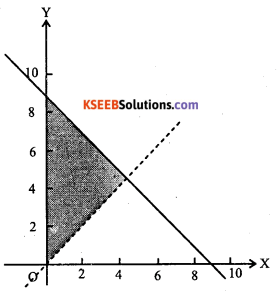(xiii) Consider x + 2y≤8 …………….. (1)
Draw the graph of x + 2y = 8 by thick line. It passes through (8, 0) and (0,4).
Join these points, put x = 0 and y = 0 in (1), we get 0 + 0 < 8 which is true.
Solution set of (1) is containing the origin.
Consider 2x + y≤8 …………….. (2)
Draw the graph of 2x + y = 8 by thick line. It passes through (4, 0) and (0, 8)
Join these points, put x = 0 and y = 0 in (2), we get 0 + 0 < 8 is true.
Solution set of (2) is containing the origin. Since x > 0, y > 0, every point in the shaded region in the first quadrant, including the points on the lines, represents the solution of the given system of inequations.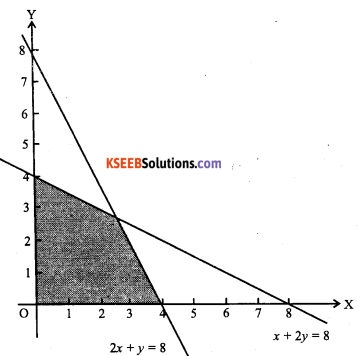(xiv) Consider 3x+4y≤60 …………. (1)
Draw the graph of 3x+4y=60 by thick line.
It passes through (20, 0) and (0, 15). Join these points, put x = 0 and y = 0 in (1), we get 0 + 0 < 60 is true.
∴ Solution set of (1) contains (0, 0). Consider x + 3y < 30 ………………… (2)
Draw the graph of x + 3y = 30.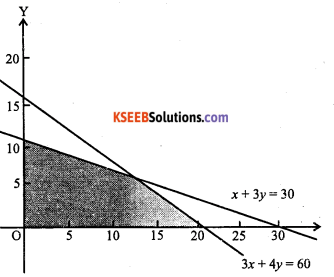It passes through (30,0) and (0,10)
Join these points, put x = 0 and y = 0 in (2), we get 0 + 0 ≤ 30 is true.
∴ Solution set of (2) contains (0, 0).
Since x ≥ 0, y ≥ 0 every point in the shaded region in the quadrant, including the points on the lines, represents the solution of the given solution of inequations.

(xv) Consider 2x + y > 4  …………. (1)
Draw the graph of 2x + y = 4 by thick line It passes through (2, 0) and (0, 4)
Join these points, put x = 0 and y = 0 in (1), we get 0 + 0 ≥ 4 is not true.
∴ Solution set of (1) does not contain (0, 0).

Consider x + y≤3 …………. (2)
Draw the graph of x + y = 3, by thick line.
It passes through (3, 0) and (0, 3).
Join these points, put x = 0 and y = 0 in (2), we get 0 + 0 < 3, which is true

Solution set of (2) contains (0, 0) Consider 2x-3y≤ 6 …………. (3)
Draw the graph of 2x – 3y = 6 by thick line It passes through (3, 0) and (0, -2)
Join these points, put x = 0 and y = 0 in (3), we get 0 – 0 < 6, which is true.
∴ Solution set of (3) contains (0, 0)
Hence, the shaded region represents the solution set of the given system of inequations.(xvi) Consider x – 2y≤3 ………….. (1)
Draw the graph of x -2y = 3 by thick line.
It passes through $$(3,0) \text { and }\left(0,-\frac{3}{2}\right)$$
Join these points, put x = 0 and y = 0 in (1), we get 0 – 0 < 3, which is true.
∴Solution of (1) contains the origin.
Consider 3x + 4y > 12 …………. (2)
Draw the graph of 3x + 4y = 12 by thick line.
It passes through (4, 0) and (0, 3).
Join these points, put x = 0 and y = 0 in (2) we get 0 + 0 > 12 which is false.
∴ Solution set of (2) does not contain the origin.
Consider y>1   ………….. (3)
Draw the graph of y = 1 clearly solution set of (3) does not contain (0, 0).
Since x > 0, every point in the shaded region in the first quadrant, including the points on the lines, represents the solution of the given system of inequations.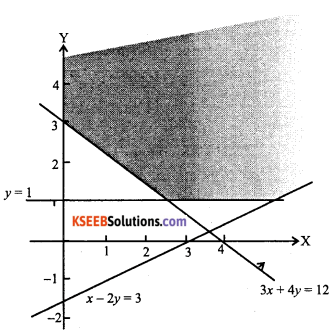(xvii) Consider 4x+3y≤60 ……….. (1)
Draw the graph of 4x+3y = 60 by the thick line.
It passes through (15, 0) and (0, 20)
Join these points, put x = 0 and y = 0 in (1), we get 0 – 0 ≤ 60 ,which is true.
∴ Solution set of (1) contains(0,0)
Consider y ≥2x ……………. (2)
Draw the graph of y = 2x by thick line
It passes through (0, 0) and (1,2)
Join these points, clearly solution set of (2) is not containing (1,1)
Consider x ≥ 3 ……………. (3)
Draw the graph of x = 3 clearly solution set of (3) does not contain the origin.
Since y > 0, every point in the shaded region in the first quadrant, including the points on the lines, represents the solution of the given system of inequations.(xviii) Consider 3x+2y≤150 ……………. (1)
Draw the graph of 3x+2y = 150
It passes through (50, 0) and (0, 75) put x = 0 and y = 0 in (1), we get 0 +0 < 150, which is true.
∴ Solution set of (1) contains (0, 0)
Consider x + 4y < 80 ……………. (2)
Draw the graph of x + 4y = 80.
It passes through (80, 0) and (0, 20).
Join these points, put x = 0 and y = 0 in (2), we get 0 + 0 < 80, which is true.
∴ Solution set of (2) contains (0, 0) Consider x < 15 ……………. (3)
Clearly solution set of (3) does not contain the origin since x ≥ 0, y ≥ 0, every point in the shaded region in the first quadrant, including the points on the lines, represents the solution of the given system of inequations.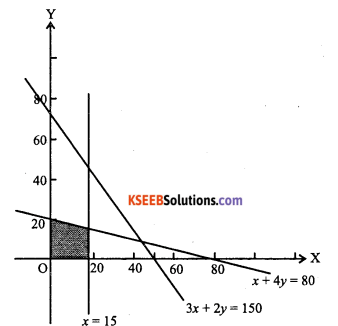(xix) Consider x+2y≤10 …………(1)
Draw the graph of x+2y=10
It passes through (10, 0) and (0, 5) put x – 0 and y = 0 in (1), we get 0 + 0 < 10, which is true.
∴ Solution set of (1), contain (0, 0)
Consider x + y > 1  ………… (2)
Draw the graph of x + y = 1
It passes through (1,0) and (0, 1) put x = 0 and y = 0 in (2), we get 0 + 0 > 1, which is false.∴ Solution set of (2) is not containing the origin
Consider x – y < 0 …………  (3)
Draw the graph of x – y = 0.
It passes through (0, 0) and (1, 1) put x = 2 and y = 0 in (3) we get
2 – 0 < 0 which is false
∴ Solution set of (3) does not contain (2, 0)
Since x > 0, y > 0, every point in the shaded region in the first quadrant, including the points on the lines, represents the solution of the given system of inequations.

Question 34.
Solve the Equations.
(i) 2≤3x-4≤5
(ii) -8≤ 5x – 3< 7
(iii) 6 < -3(2JC – 4) < 12
(iv) $$-3 \leq 4-\frac{7 x}{2} \leq 18$$
(v) $$-5 \leq \frac{5-3 x}{2} \leq 8$$
(vi) $$-15<\frac{3(x-2)}{5} \leq 0$$
(vii) $$7 \leq \frac{3 x+11}{2} \leq 11$$
(viii) $$-12<4-\frac{3 x}{-5} \leq 2$$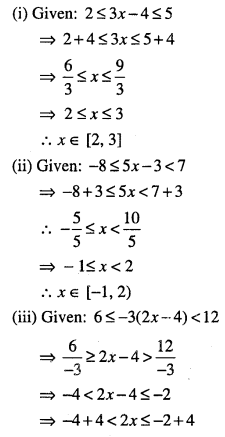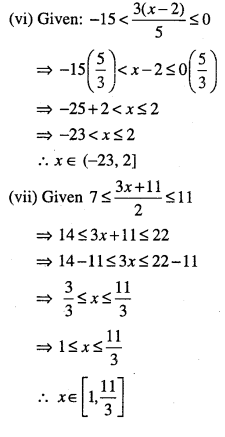Question 35.
Solve the inequalities and represent the solution graphically on number line:
(i) 5x+ 1 > -24, 5x-1 < 24
(ii) 3x – 7 < 5 + x, 11 – 5x≤1
(iii) 3x – 7 > 2(x – 6), 6 – x > 11. – 2x
(iv) 2(x – 1) < x + 5,3(x + 2) > 2 – x
(v) 5(2x – 7) – 3(2x+ 3)≤0, 2x + 19≤6x + 47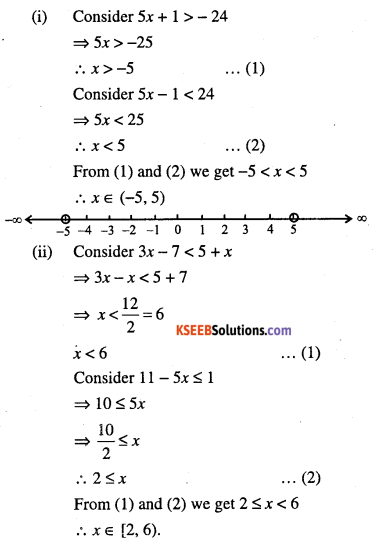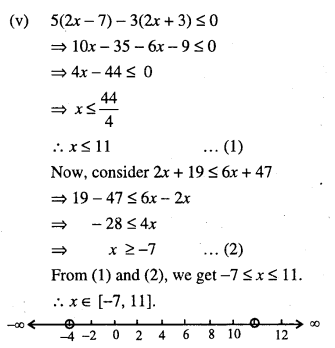Question 36.
In an experiment, a solution of hydrochloric acid is to be kept between 30° and 35° Celsius. What is the range of temperature in degree Fahrenheit if conversion formula is given by $$C=\frac{5}{9}(F-32)$$, where C and F represents temperature in degree Celsius and degree Fahrenheit, respectively.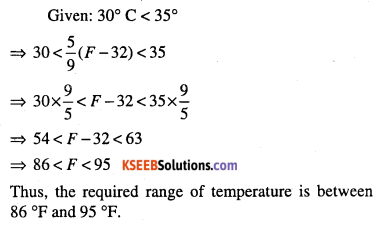Question 37.
A solution is to be kept between 68 °F and 77 °F. What is the range in temperature in degree Celsius (C) if the Celsius/Fahrenheit (F) conversion formula is given by
$$F=\frac{9}{5} C+32$$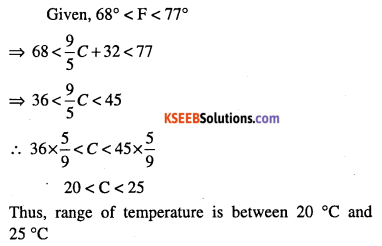Question 38.
A solution of 8% boric acid to be diluted by. adding a 2% boric acid solution to it. The resulting mixture is to be more than 4% but less than 6% boric acid. If we have 640 liters of the 8% solution, how many liters of the 2% solution will have to be added?
Let x be the number of liters of 2% boric acid solution.
∴ Total mixture = (640 + x ) liters.
$$\Rightarrow \frac{2 x}{100}+\frac{8}{100}(640)>\frac{4}{100}(640+x)$$
⇒ 2x+ 5120 >2560+ 4x
⇒ 5120-2560 >4x-2x
⇒ 2x< 2560
⇒ x< 1280 ………. (1)
Also, 2% of x+ 8% of 640 < 6% of (640 + x)
$$\Rightarrow \frac{2 x}{100}+\frac{8}{100}(640)<\frac{6}{100}(640+x)$$
⇒ 2x + 5120 < 3840 + 6x
⇒ 5120-3840 <6x-2x
⇒ 1280 < 4x
⇒ 320< x …………….. (2)
From (1) and (2), we get 320 < x< 1280Question 39.
A manufacturer has 600 liters of 12% solution of acid. How many liters of a 30% acid solution must be added to it so that acid content in the resulting mixture will be more than 15% but less than 18%?
Let x liters of 30% acid solution is required to be added.
Then, total mixture = (x + 600) liters.
∴ 30% of x + 12% of 600 > 15% of (x + 600)
$$\Rightarrow \frac{30 x}{100}+\frac{12}{100}(600)>\frac{15}{100}(x+600)$$
⇒ 30x + 7200 > 15x + 9000
⇒ 15x> 9000 -7200= 1800
⇒ x > 120 …………………… (1)
Also 30% of x + 12% 600 < 18% of (x + 600)
$$\Rightarrow \frac{30 x}{100}+\frac{12}{100}(600)<\frac{18}{100}(x+600)$$
⇒ 30x + 7200 < 18x+ 10800
⇒ 12x < 10800 – 7200 = 3600
⇒ x <300 …………. (2)
From (1) and (2) we get 120 < x < 300
Thus, the number of liters of the 30% solution of acid will have to be more than 120 liters but less than 300 liters.

Question 40.
How many liters of water will have to be added to 1125 liters of the 45% solution of acid so that the resulting mixture will contain more than 25% but less than 30% acid content?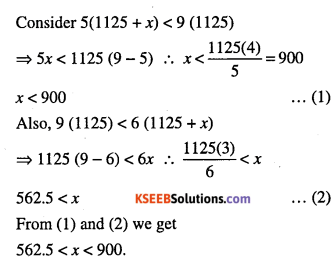IQ of a person is given by the formula $$I Q=\frac{M A}{C A} \times 100$$ where MA is mental age and CA CA is a chronological age. If 80 < IQ < 140 for a group of 12 years old children, find the range of their mental age.